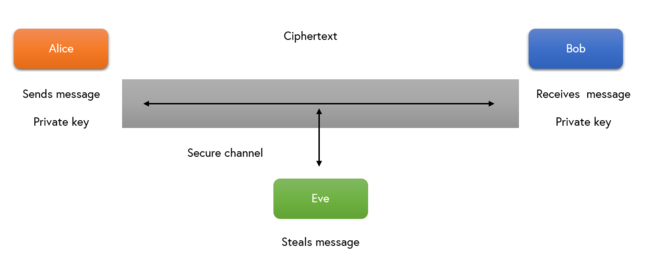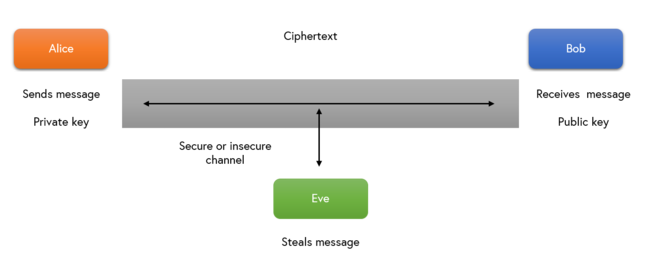1.7

# Cryptography recap

Encryption and hashing was explored extensively in the short course An Introduction to Cryptography. Here, we provide a quick recap.

Generally, we could say that the purpose of cryptography is to keep the system’s data/information secret and secure. There are two main encryption methods: symmetric and asymmetric cryptography.

• Symmetric key encryption makes use of a single key for both the encryption and decryption process. Note that this key needs to be kept secret from third parties and should also be exchanged and shared only between the valid communication parties through a secure communication channel.
• Asymmetric or public key encryption makes use of two keys. The first key is called a public key and can be known to anyone and is used for the encryption. The second key, known as the private key, should be kept secret and is used for the decryption of the exchanged data. Note that in this approach, the public key does not necessarily need to be exchanged over a secure channel.

To have a better understanding of the two methods, we present the encryption and decryption process taking place in each of them in the form of algorithms, involving Alice, Bob and Eve.

### Symmetric cryptography algorithm

The figure below demonstrates a typical communication that uses symmetric cryptography (click image to zoom).Algorithm steps:

• Alice and Bob agree on the private key
• Alice passes the key to Bob
• Alice creates ciphertext using the private key:

ciphertext = encryptionkey (message)

• Alice sends it through the communication channel
• Bob receives the ciphertext and decrypts it using the private key:

message = decryptionkey (ciphertext)

• Bob receives the original message/plaintext

### Asymmetric cryptography algorithm

The figure below demonstrates a typical communication that uses asymmetric cryptography (click image to zoom):Algorithm steps

• Alice creates her private key and a public key (similarly for Bob)
• Alice hides her private key and sends out a copy of the public key to Bob (similarly for Bob)
• Bob creates the ciphertext using Alice’s public key (similarly for Alice):

ciphertext = encryptionAlice public key (message)

• Bob sends it through the communication channel (similarly for Alice)
• Alice receives the ciphertext and decrypts it using her private key (similarly for Bob):

message = decryptionAlice private key (ciphertext)

• Alice receives Bob’s original message/plaintext (similarly for Bob)

### Hashing

We could define a hashing function as a special mathematical function, denoted by H, performing one-way encryption. The input is a plain message and the output is an alphanumeric hash value. The mathematical formula of a hash function is as follows:

*H(message) = hash*


It is worth pointing out that a hash function needs to satisfy certain properties in order to be characterised as a strong and well-defined hash function. These properties are:

• Pre-image resistant: This property ensures that given a hash value, the computation of the input message, such that hash= H(message), is hard.
• Avalanche effect: Any small change to the message results in a totally different hash output.
• Second-pre-image resistant: Given a message, it is hard to find message’ (different from message) such that H(message) = H(message’)
• Collision resistant: It is hard to find message and message’ such that H(message) = H(message’).

The satisfaction of these properties could guarantee that the plain message used in the hash function cannot be easily retrieved by unauthorised parties.

### Conclusion

The application of the security principles and methods described here and on the next step could be a good starting point to effectively secure a computer system that is under development.